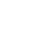# Code Challenges #2: 0's surrounded by 1's

This week in Code Challenges, I was asked to write an algorithm to find the longest sequence of 0's surrounded by 1's.# Given a non-negative number, find the longest sequence of 0s surrounded by 1s in the binary representation of that number

So for example, given `5 (base 10) == '101' (binary, base 2)`, the longest sequence of 0's surrounded by 1's is 0. Here are some more examples.

``````100           => 0 // because the 0s are not boxed in by 1s.
1000001001    => 5 // because the max sequence between the two found here is 5
001           => 0 // again, because the 0s are not boxed by 1s.
1             => 0
0             => 0``````

Here's what I got as my solution for this problem.

``````function solution(N) {
// convert the number to binary representation
let text = N.toString(2);
let index = 0;
let max = 0;

// Iterate over the elements
while (index < text.length - 1) {
// If the current element is a 1
if (text.charAt(index) == "1") {
// Then look down the line and see if there's a 1 down there somewhere.
if (text.substring(index + 1).indexOf("1") !== -1) {
// If there is, then calculate the new max as the distance from this
// current index to that 1 down the line.
let distance = text.substring(index + 1).indexOf("1");
max = Math.max(max, distance);
// Then, since we know that there's only going to be 0s in between
// our current index and the 1 that we found, let's just jump to
// that next 1 and continue from there.
index = index + distance + 1;
}

// Otherwise, just increment to the next element
else {
index++;
}
}
else {
index++;
}
}
return max;
}

solution(5);``````

So really, it can be conceptualized that we'll only take action if the current element we're looking at is a 1. And if it is, then we'll see if there's another one somewhere yonder and get the new max if there is.

This solution solves the problem in O(log N) time by (in good scenarios) cutting down the search space in large chunks each time like a divide and conquer algorithm.

Can you suggest any improvements to this algorithm? Share your solution in the comments below!

### Discussion

Liked this? Sing it loud and proud 👨‍🎤.

### Stay in touch!Khalil Stemmler,
Developer Advocate @ Apollo GraphQL ⚡

Khalil is a software developer, writer, and musician. He frequently publishes articles about Domain-Driven Design, software design and Advanced TypeScript & Node.js best practices for large-scale applications.

View more in Code Challenges## You may also enjoy...

A few more related articlesBooks that Developers Should Read in 2020 [List]
These books “are basically cheat codes” for leveling up your skills and knowledge as a developer.There Is No Dominant Paradigm | Software Professionalism
Functional programming is not the end to all of our problems. Quality software is all three paradigms.Name, Construct & Structure | Organizing Readable Code - Part 1
Naming files & folders well, using well-understood technical constructs and strategically organizing files well are three ways to ...Over \$85 billion spent on fixing bad code [bootcamps, junior devs, JavaScript, and software design principles]
More and more money is being spent by companies on maintaining bad JavaScript code. Here's where I think our industry needs to cha...Want to be notified when new content comes out?

Join 5000+ other developers learning about Domain-Driven Design and Enterprise Node.js.

I won't spam ya. 🖖 Unsubscribe anytime.